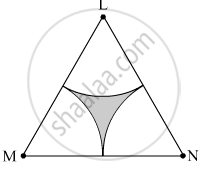Share

# Δ Lmn is an Equilateral Triangle. Lm = 14 Cm. as Shown in Figure, Three Sectors Are Drawn with Vertices as Centres and Radius 7 Cm. Find, Area of the Shaded Region. - Geometry

ConceptAreas of Sector and Segment of a Circle

#### Question

$∆$ LMN is an equilateral triangle. LM = 14 cm. As shown in the figure, three sectors are drawn with vertices as centers and radius 7 cm.
Find, Area of the shaded region.#### Solution

∆LMN is an equilateral triangle.
∴ LM = MN = LN = 14 cm
∠L = ∠M = ∠N = 90º

Area of the shaded region = Area of ∆LMN − Total area of all the three sectors = 84.87 − 77.01 = 7.86 cm2

Is there an error in this question or solution?

#### APPEARS IN

Balbharati Solution for Balbharati Class 10 Mathematics 2 Geometry (2018 to Current)
Chapter 7: Mensuration
Practice set 7.3 | Q: 13.4 | Page no. 155
Solution Δ Lmn is an Equilateral Triangle. Lm = 14 Cm. as Shown in Figure, Three Sectors Are Drawn with Vertices as Centres and Radius 7 Cm. Find, Area of the Shaded Region. Concept: Areas of Sector and Segment of a Circle.
S